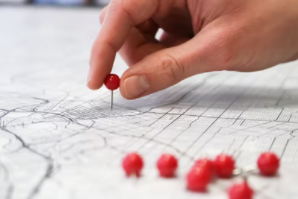## Another Brain Teaser

You drive from Point A to B at 60mph and then stop at a diner in B for 30 minutes. You then drive from B to C at a speed of 30mph. When you reach C, your watch tells you that you left point A four hours ago. Looking over the map, you realize that the distance from B to C is 1/4 of the distance from A to B. How many minutes did it take you to drive from point B to C?Hint
Note that 60mph = 1mi/min and 30mph = 0.5mi/min
Hint 2
Solve for time (t) it takes to drive from B to C. Let $$t_0$$ be the time it takes to drive from A to B.
$$t_{0}+t=240min-30min=210min$$$Solve for time (t) it takes to drive from B to C. Let $$t_0$$ be the time it takes to drive from A to B. Note that 60mph = 1mi/min and 30mph = 0.5mi/min $$t_{0}+t=240min-30min=210min$$$
$$t_{0}=210min-t$$$The distance from A to B = 4x distance from B to C. $$1mi/min(210min-t)=4(t)(0.5\frac{mi}{min})$$$
Solving for (t):
$$210min=3t$$$$$t=70\: min$$$
70 min